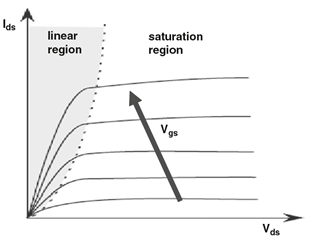If you have a related question, please click the "Ask a related question" button in the top right corner. The newly created question will be automatically linked to this question.

# CSD19538Q3A: Saturation or Linear Zone?

Part Number: CSD19538Q3A

Hi,

I have a question regarding the area in which a MOSFET works when it is used in ON / OFF switching.

I believe the mosfet should be used in the saturation zone when ON (therefore with with R between drain and source = RDSon) as if used, instead, in the linear zone we would have had a greater R between drain and source and consequently a greater dissipation, while I have doubt instead that it could be used in a linear (or resistive) area because the dissipation on the mosfet is lower and for this reason the mosfet uses the saturation condition for the signal amplifications. But I am not sure about this.

Thank you!

Antonio

• Hi Antonio,

Thanks for the inquiry. The operating regions of a MOSFET are defined as follows:

– linear region, where Vds << Vgs − Vth (constant on resistance mode, power loss = I^2 x Ron)

– saturation region, where Vds > Vgs − Vth (linear mode, power loss = Vds x Ids)I hope this answers your questions and clarifies MOSFET modes of operation.

Thanks,

John Wallace

TI FET Applications

• Hi John,

Thank you for the answer, I only have a little doubt left:
The area where you should use the MOSFET in switching in the ON state is therefore that of saturation, where we have a lower dissipation on the mosfet (where the R between drain and source is the lowest possible), right?
BR,

Antonio

• Hi Antonio,

Sometimes the terminology can be confusing. As shown in my earlier response, the MOSFET is on (enhanced) when it is operating in the linear region or constant resistance mode. There is a linear relationship between Vds and Ids: Vds = Ids x Ron and the power dissipation is Ids^2 x Ron.

In the saturation region, often referred to as linear mode, Ids is flat as Vds increases as shown in the plot and the power dissipation is Ids x Vds. The MOSFET is saturated and increasing Vds does not cause an increase of Ids.

TI power MOSFETs have on resistance specified in the linear region at VGS >> VTH.

Thanks,

John